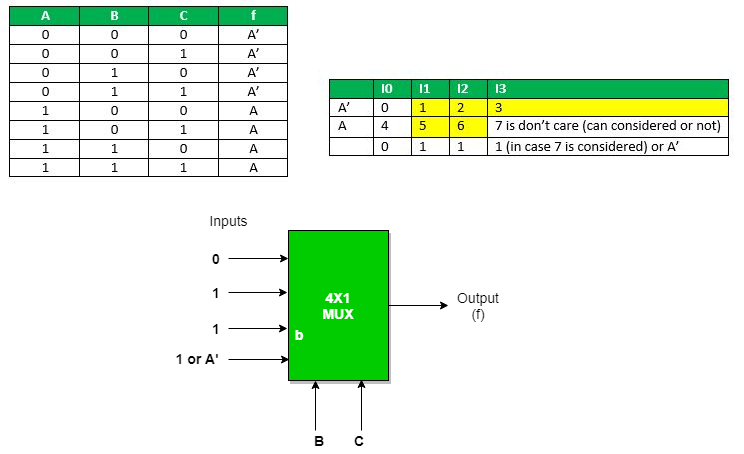# Multiplexers in Digital Logic

• Difficulty Level : Medium
• Last Updated : 23 Dec, 2020

It is a combinational circuit which have many data inputs and single output depending on control or select inputs.​ For N input lines, log n (base2) selection lines, or we can say that for 2n input lines, n selection lines are required. Multiplexers are also known as “Data n selector, parallel to serial convertor, many to one circuit, universal logic circuit​”. Multiplexers are mainly used to increase amount of the data that can be sent over the network within certain amount of time and bandwidth.

Attention reader! Don’t stop learning now.  Practice GATE exam well before the actual exam with the subject-wise and overall quizzes available in GATE Test Series Course.

Learn all GATE CS concepts with Free Live Classes on our youtube channel.Now the implementation of 4:1 Multiplexer using truth table and gates.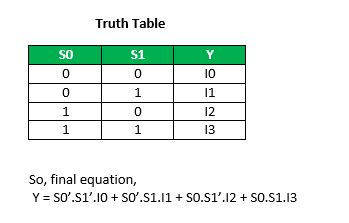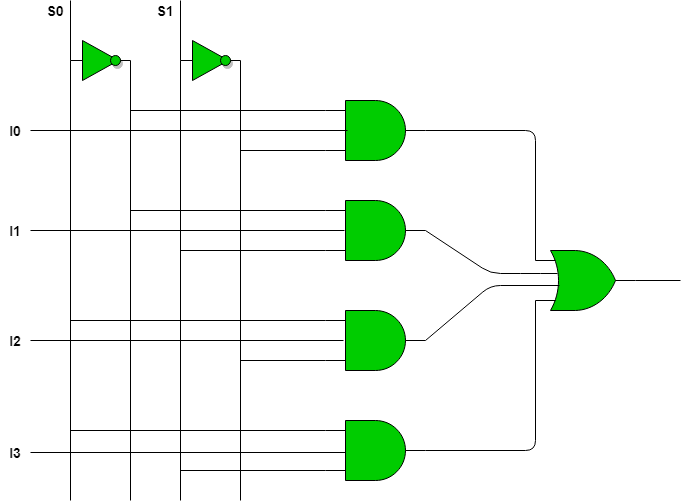Multiplexer can act as universal combinational circuit. All the standard logic gates can be implemented with multiplexers.

a) Implementation of NOT gate using 2 : 1 Mux

NOT Gate :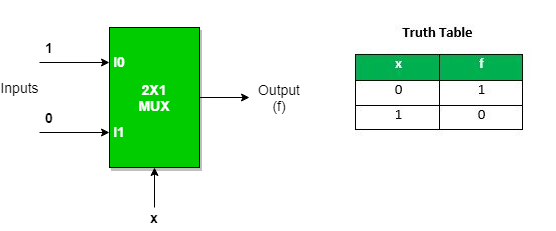We can analyze it
Y = x’.1 + x.0 = x’
It is NOT Gate using 2:1 MUX.
The implementation of NOT gate is done using “n” selection lines. It cannot be implemented using “n-1” selection lines. Only NOT gate cannot be implemented using “n-1” selection lines.

b) Implementation of AND gate using 2 : 1 Mux

AND GATE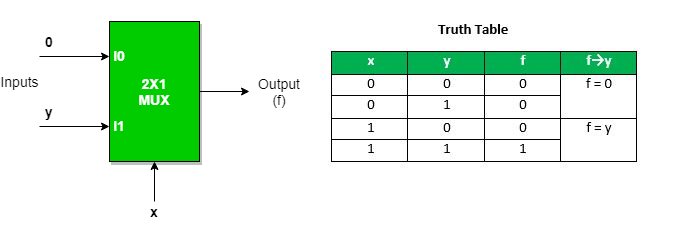This implementation is done using “n-1” selection lines.

c) Implementation of OR gate using 2 : 1 Mux using “n-1” selection lines.

OR GATE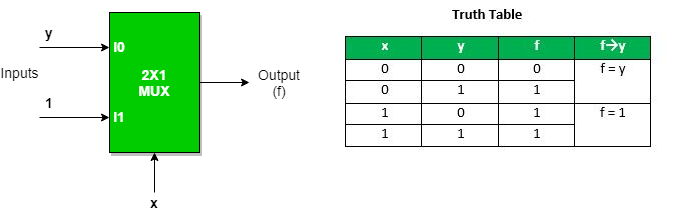Implementation of NAND, NOR, XOR and XNOR gates requires two 2:1 Mux. First multiplexer will act as NOT gate which will provide complemented input to the second multiplexer.

d) Implementation of NAND gate using 2 : 1 Mux

NAND GATE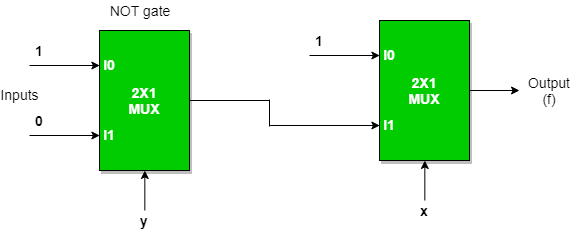e) Implementation of NOR gate using 2 : 1 Mux

NOR GATEf) Implementation of EX-OR gate using 2 : 1 Mux

EX-OR GATE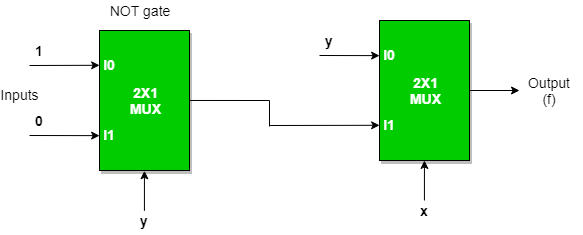g) Implementation of EX-NOR gate using 2 : 1 Mux

EX-NOR GATE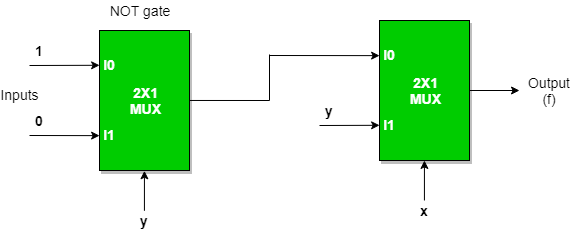Implementation of Higher order MUX using lower order MUX

a) 4 : 1 MUX using 2 : 1 MUX

Three(3) ​2 : 1 MUX are required to implement 4 : 1 MUX.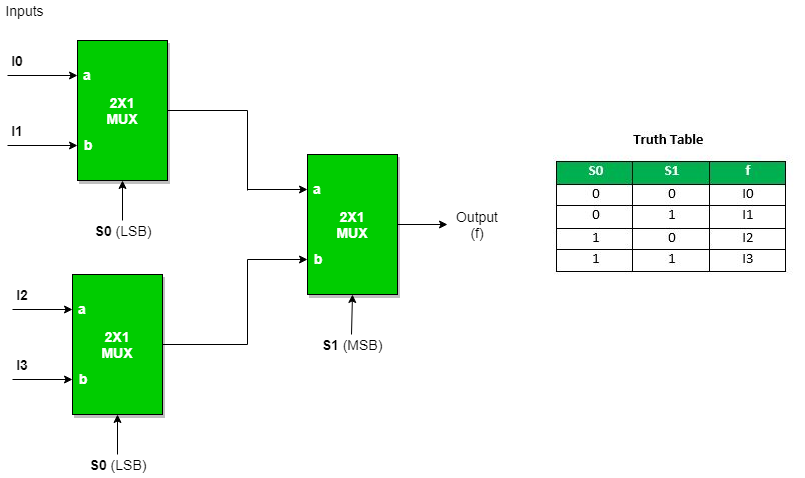Similarly,

While 8 : 1 MUX require seven(7) ​2 : 1 MUX, 16 : 1 MUX require fifteen(15) ​2 :1 MUX, 64 : 1 MUX requires sixty three(63)​ 2 : 1 MUX.
Hence, we can draw a conclusion,
2n : 1 MUX requires (2^n – 1) 2 : 1 MUX.

b) 16 : 1 MUX using 4 : 1 MUX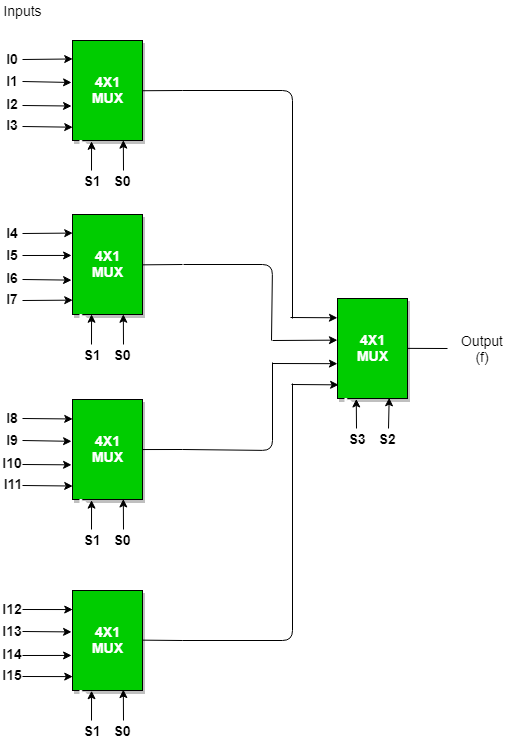In general, to implement B : 1 MUX using A : 1 MUX , one formula is used to implement the same.
B / A = K1,
K1/ A = K2,
K2/ A = K3

………………

KN-1 / A = KN = 1 (till we obtain 1 count of MUX).

And then add all the numbers of MUXes = K1 + K2 + K3 + …. + KN
For example​ : To implement 64 : 1 MUX using 4 : 1 MUX
Using the above formula, we can obtain the same.
64 / 4 = 16
16 / 4 = 4
4 / 4 = 1 (till we obtain 1 count of MUX)
Hence, total number of 4 : 1 MUX are required to implement 64 : 1 MUX = 16 + 4 + 1 = 21.

An example to implement a boolean function if minimal and don’t care terms are given using MUX​.
f ( A, B, C) = Σ ( 1, 2, 3, 5, 6 ) with don’t care (7) using 4 : 1 MUX using as
a) AB as select : ​Expanding the minterms to its boolean form and will see its 0 or 1 value in Cth place so that they can be placed in that manner.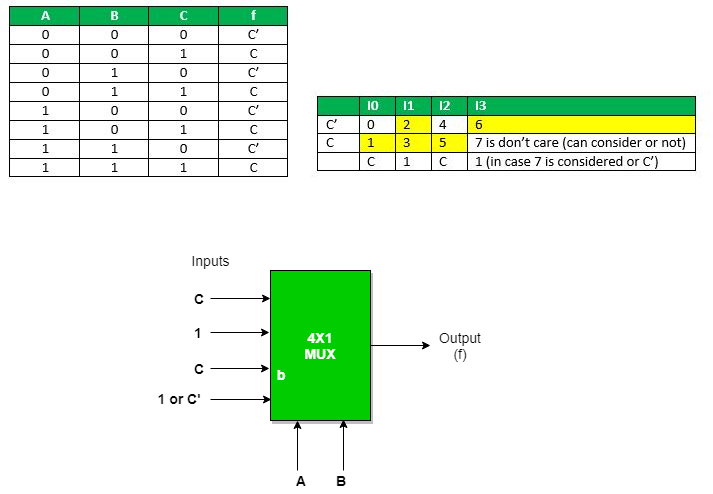b) AC as select : Expanding the minterms to its boolean form and will see its 0 or 1 value in Bth place so that they can be place in that manner.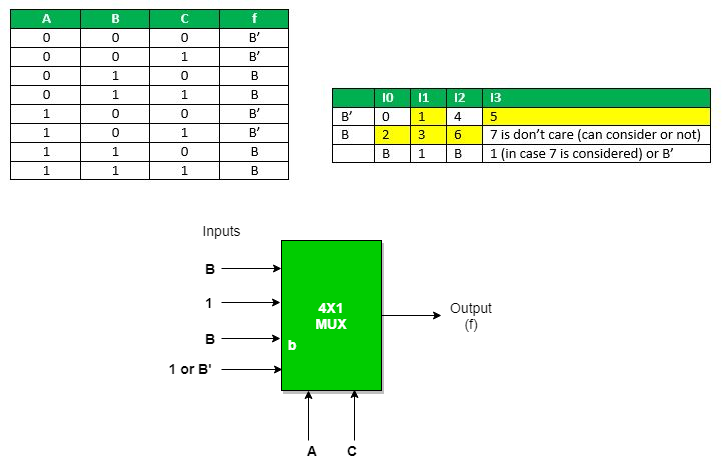c) BC as select : ​Expanding the minterms to its boolean form and will see its 0 or 1 value in Ath place so that they can be place in that manner.